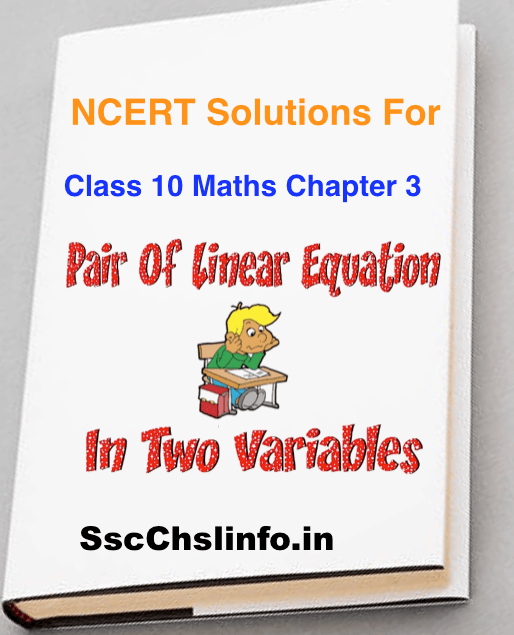# NCERT Solutions For Class 10 Maths Chapter 3 in English Medium

NCERT Class 10 maths Books are very important for students who want to get good marks in their exams. In this post, we published NCERT Solutions for class 10 Maths chapter 3. Name of class 10 Maths chapter 3 is Pair of linear equations in two variables. Class 10 Students have faced many problems to solve exercise question so our main aim on this site is to provide maximum solution for each and every kind questions. you can download NCERT Solutions For Class 10 Maths special class notes collection. you will enjoy it.

In NCERT class 10 Maths chapter 3 have following exercise (3.1 ,3.2,3.3 ,3.4,3.5,3.6, , 3.7) in English medium in PDF format. In NCERT Solutions Class 10 Maths we have solved each and every question only for you.

all the exercises are available in a form of class notes from one reputed school or the solution is written by hand. The NCERT Solutions for class 10 Maths chapter 3 is specially designed for CBSE exam Pattern with the latest CBSE Syllabus.## Pair of Linear Equations in Two Variables Solutions of Class 10th Math

All solution is 100% correct so you can download our NCERT Solutions for Class 10 Maths Chapter 3 with detail explanation and It was solved by our  Maths teachers with full dedication and hard working. We present step by step solutions for all the questions given in class 10 maths textbook according to the CBSE Board guidelines from the newest NCERT book for class 10 maths.

### Download Exercises Wise NCERT Solutions in Chapter 3 in math book

• 3.3 Graphical Method of Solution of a pair of Linear Equations, Click Here
• 3.4 Algebraic Methods of Solving a Pair of Linear Equations Click Here

3.4.1 Substitution Method

3.4.2 Elimination Method

3.4.3 Cross-Multiplication

We strictly advised the students that they should also prepare and study all the solved previous examples paper which provided in the textbook to clear your basic concepts on Pair of Linear Equations in Two Variables.

Our website provides downloadable the free PDF Link to Chapter 3 Pair of Linear Equations in Two Variables for the student’s exam preparation.

You don’t need to download full solution. you can directly view it from below section.

Trending article:Written by

##### Ompal Rao

I love to share the latest information about govt job & Previous year question paper with answer. NCERT Solutions For Class 11 Maths & NCERT Class 10 Math Solution

#### One thought on “NCERT Solutions For Class 10 Maths Chapter 3 in English Medium”

1.hitesh says:

This helps. Thanks!

This site uses Akismet to reduce spam. Learn how your comment data is processed.